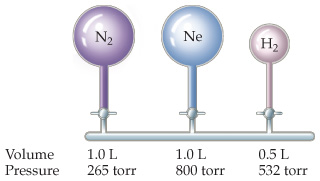# Problem: Consider the arrangement of bulbs shown in the drawing. Each of the bulbs contains a gas at the pressure shown.What is the pressure of the system when all the stopcocks are opened, assuming that the temperature remains constant? (We can neglect the volume of the capillary tubing connecting the bulbs.)

###### FREE Expert Solution

We are asked to determine the pressure of the system when all the stopcocks are opened, assuming that the temperature remains constant.According to Boyle’s Law, an inverse relationship exists between pressure and volume assuming the number of molecules (n) and the temperature (T) are both constant.

The relationship for Boyle’s Law can be expressed as follows:

where:

P1 and V1 are the initial pressure and volume values

P2 and V2 are the values of the pressure and volume of the gas after the change.

94% (117 ratings)###### Problem Details

Consider the arrangement of bulbs shown in the drawing. Each of the bulbs contains a gas at the pressure shown.

What is the pressure of the system when all the stopcocks are opened, assuming that the temperature remains constant? (We can neglect the volume of the capillary tubing connecting the bulbs.)Frequently Asked Questions

What scientific concept do you need to know in order to solve this problem?

Our tutors have indicated that to solve this problem you will need to apply the The Ideal Gas Law Derivations concept. You can view video lessons to learn The Ideal Gas Law Derivations. Or if you need more The Ideal Gas Law Derivations practice, you can also practice The Ideal Gas Law Derivations practice problems.

What professor is this problem relevant for?

Based on our data, we think this problem is relevant for Professor Davis' class at UCF.